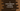# C program to count even and odd numbers in a user input array# Count odd and even number in an array in C:

In this C programming tutorial, we will learn how to count the odd and even numbers in an array. The program will populate the array taking inputs from the user and it will print out the even and odd count.

## Algorithm :

The following algorithm we will use in the program :

1. Initialize two integer variables as 0 to store the odd and even number count.
2. Ask the user to enter the size of the array.
3. Create one_ integer array_ as the same size that the user has entered.
4. Using one loop, take the input of the array from the user one by one.
5. Iterate through the array one more time using one loop.
6. Check for each number if it is an even number or odd number. If the number is even, increment the even count by 1 and if the number is odd, increment the odd count by 1.
7. After the loop is completed, print out the even count and odd count to the user.

We will learn to solve this problem by using a for loop and by using a while loop.

### C program to count odd and even numbers in an array using a for loop :

``````#include <stdio.h>
int main()
{
//1
int oddCount = 0;
int evenCount = 0;
int sizeOfArray;
int i;
//2
printf("Enter the size of the array : ");
scanf("%d", &sizeOfArray);
//3
int array[sizeOfArray];
//4
for (i = 0; i < sizeOfArray; i++)
{
printf("Enter item for position %d : ", i);
scanf("%d", &array[i]);
}
//5
for (i = 0; i < sizeOfArray; i++)
{
//6
if (array[i] % 2 == 0)
{
evenCount++;
}
else
{
oddCount++;
}
}
//7
printf("Even count : %d\n", evenCount);
printf("Odd count : %d\n", oddCount);
return 0;
}``````

### Explanation :

The commented numbers in the above program denote the step numbers below :

1. Create four integer variables: oddCount to store the total odd number count, evenCount to store the total even number count,sizeOfArray to store the size of the array and_ i_ to use in the loop.
2. Ask the user to enter the size of the array. Read it using scanf and store it in sizeOfArray.
3. Create one integer array array of size sizeOfArray.
4. Run one for loop to read the contents of the array. Read each item one by one and store it in the array.
5. Iterate the array one more time using a for loop.
6. Check for each item if it is even or odd. If even, increment the variable evenCount by 1, else increment oddCount by 1.
7. Finally, print out the even and odd count variables to the user.

## Sample Output :

``````Enter the size of the array : 5
Enter item for position 0 : 1
Enter item for position 1 : 12
Enter item for position 2 : 23
Enter item for position 3 : 44
Enter item for position 4 : 45
Even count : 2
Odd count : 3

Enter the size of the array : 3
Enter item for position 0 : 1
Enter item for position 1 : 3
Enter item for position 2 : 5
Even count : 0
Odd count : 3``````

### C program to count odd and even numbers in a user given array using a while loop :

``````#include <stdio.h>
int main()
{
int oddCount = 0;
int evenCount = 0;
int sizeOfArray;
int i;
printf("Enter the size of the array : ");
scanf("%d", &sizeOfArray);
int array[sizeOfArray];
while(i < sizeOfArray)
{
printf("Enter item for position %d : ", i);
scanf("%d", &array[i]);
i++;
}
i = 0;

while(i < sizeOfArray)
{
if (array[i] % 2 == 0)
{
evenCount++;
}
else
{
oddCount++;
}
i++;
}
printf("Even count : %d\n", evenCount);
printf("Odd count : %d\n", oddCount);
return 0;
}``````

As you can see that this program is the same as the above one. The only difference is that we are using one while loop instead of a for loop.

The output for this program is the same as the above.

The above examples are also available here on Github.

### Conclusion :

We have learned two different ways to solve this problem: by using a for loop and by using a while loop. Try to run the examples above and drop one comment below if you have any queries.